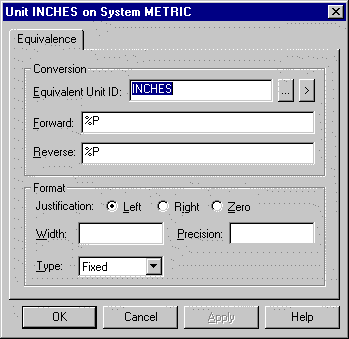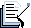### 9. Define an Equivalent Unit

Use the Unit on System dialog box to define (or edit) the information for an equivalent unit.

1. Double-click Measurement Units in the left pane of the Workbench.

The Measurement Unit–Configuration window opens.

1. Do one or the following:

Option 1

1. Select Edit on the menu bar.

2. Select Unit Equivalence.

Option 2

Press Ctrl+E on the keyboard.

Result: The Unit on System dialog box opens.Note: When you use %P as the conversion equation in a measurement unit equivalence, the display format fields such as Width, Precision, Type and Justification will be used instead because a %P requires no conversion.

You can edit any of the following fields in the right pane or in the dialog box:

 Equivalent Unit ID Enter the Unit ID you want to use when the measurement system is active. The Unit ID may or may not match any other Unit IDs in the configuration. Forward Equation Enter the equation to be used by Point Management to convert the base value to its equivalent in this measurement system. For example, if point XYZ's base value is in inches and you want to display it in centimeters, use the forward expression %P*2.54001. If the point's base value is the same as its converted value, just put %P in the field. Reverse Equation Enter the equation to be used by Point Management to convert the equivalent in this measurement system to its base value. For example, if point XYZ's base value is in inches and its equivalent value is in centimeters, use the reverse expression %P/2.54001. If the point's base value is the same as its converted value, just put %P in the field. Justification This field is used to align the display of point value. By default, the project uses the justification you specify when you configure a point. Select the justification you want to use when displaying the point's value on CimView screens when this measurement system is active, as long as the Configured option is selected for the CimView object display properties. You can select one of the following: Left Left justifies the value display. Right Right justifies the value display. Zero Zero fills the value display. Width This field represents the number of spaces you want to dedicate to the display of the point value. By default, the project uses the display width you specify when you configure a point. If you want to use a different display width for all points that use this Unit ID, enter it in this field. Precision This field represents the number of digits to be displayed to the right of the decimal point. By default, the project uses the precision you specify when you configure a point. If you want to use a different precision for all points that use this Unit ID, enter it in this field. Type This field determines the format type used when the point value is displayed. By default, the project uses the display type you specify when you configure a point. If you want to use a different display for all points that use this Unit ID, enter it in this field. Use the drop-down list button to select one of the following: Fixed All points that use the Unit ID are displayed in fixed format using the Width and Precision information you specify. If you do not specify the Precision, the default is 6. For example, if you specify a Width of 7 and Precision of 3, the point display uses 7 places and there are 3 places after the decimal point. In this case, 10 displays as 10.000. Scientific All points that use the Unit ID are displayed in scientific format using the Width and Precision information you specify. If you do not specify the precision, the default is 6. Compact All points that use the Unit ID are displayed in Fixed or Scientific format based on Precision, which determines the exponent to start displaying in Scientific format. For example, if you specify a Precision of 5, the value 100,000 displays as 1e+005 and 10,000 displays as 10000. The Compact type also truncates trailing zeros to the right of the decimal point. For example, 10.0 displays as 10 and 10.10 displays as 10.1.
 Measurement systems and units configuration.